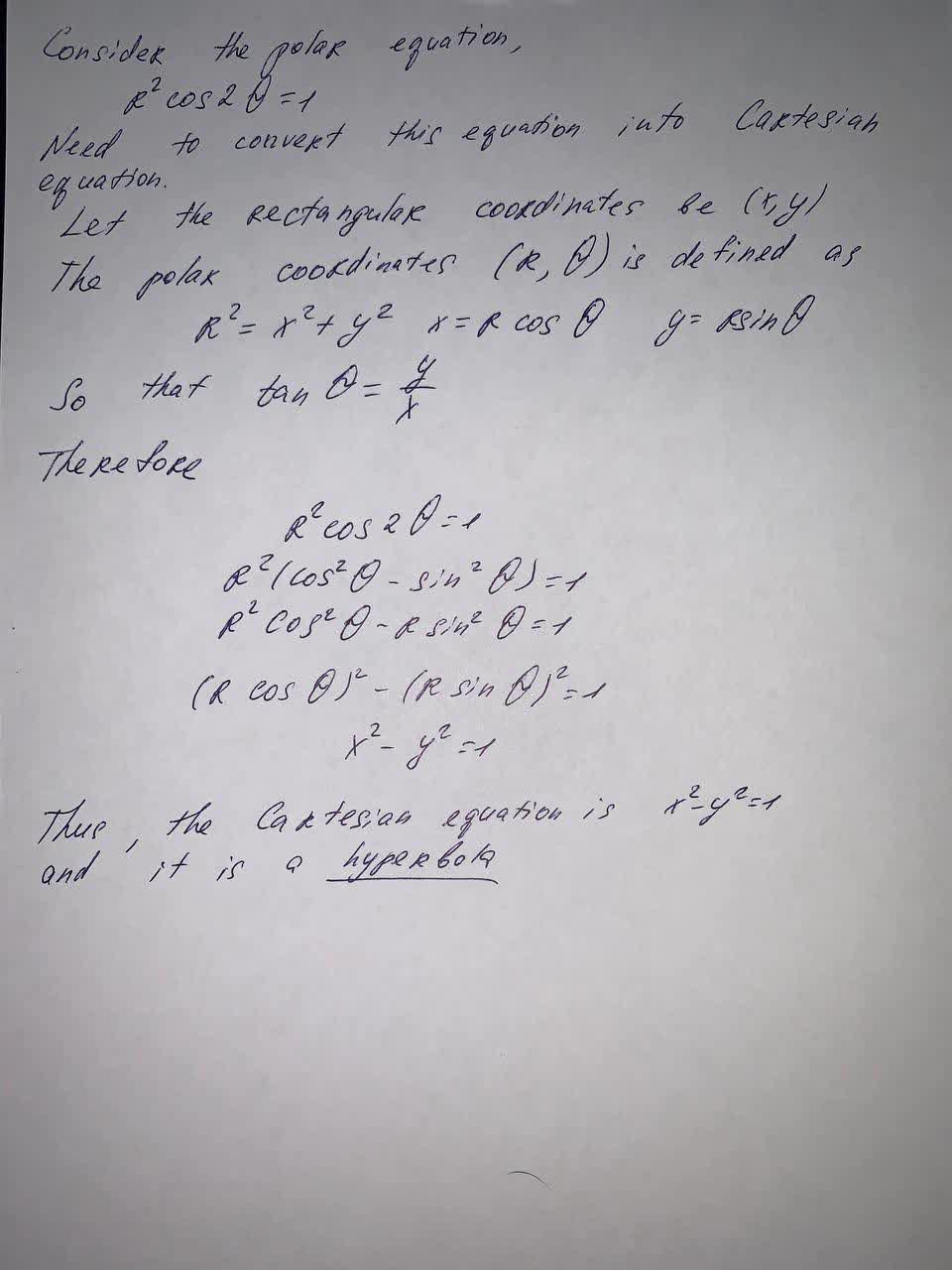Question# Find a Cartesian equation for the curve and identify it. r^2 \cos 2\theta =1

Integrals
ANSWERED$$r^2 \cos 2\theta =1$$